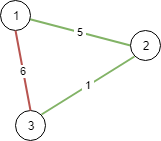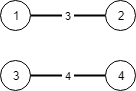1135. Connecting Cities With Minimum Cost

There are `N` cities numbered from 1 to `N`.

You are given `connections`, where each `connections[i] = [city1, city2, cost]` represents the cost to connect `city1` and `city2` together.  (A connection is bidirectional: connecting `city1` and `city2` is the same as connecting `city2` and `city1`.)

Return the minimum cost so that for every pair of cities, there exists a path of connections (possibly of length 1) that connects those two cities together.  The cost is the sum of the connection costs used. If the task is impossible, return -1.

Example 1:```Input: N = 3, connections = [[1,2,5],[1,3,6],[2,3,1]]
Output: 6
Explanation:
Choosing any 2 edges will connect all cities so we choose the minimum 2.
```

Example 2:```Input: N = 4, connections = [[1,2,3],[3,4,4]]
Output: -1
Explanation:
There is no way to connect all cities even if all edges are used.
```

Note:

1. `1 <= N <= 10000`
2. `1 <= connections.length <= 10000`
3. `1 <= connections[i], connections[i] <= N`
4. `0 <= connections[i] <= 10^5`
5. `connections[i] != connections[i]`

1135. Connecting Cities With Minimum Cost
``````struct Solution;

struct UnionFind {
parent: Vec<usize>,
n: usize,
}

impl UnionFind {
fn new(n: usize) -> Self {
let parent = (0..n).collect();
UnionFind { parent, n }
}

fn find(&mut self, i: usize) -> usize {
let j = self.parent[i];
if j == i {
i
} else {
let k = self.find(j);
self.parent[i] = k;
k
}
}

fn union(&mut self, i: usize, j: usize) {
let i = self.find(i);
let j = self.find(j);
if i != j {
self.parent[i] = j;
}
self.n -= 1;
}
}

type Connection = (i32, usize, usize);

impl Solution {
fn minimum_cost(n: i32, connections: Vec<Vec<i32>>) -> i32 {
let n = n as usize;
let mut uf = UnionFind::new(n);
let mut connections: Vec<Connection> = connections
.into_iter()
.map(|v| (v, v as usize - 1, v as usize - 1))
.collect();
connections.sort_unstable();
let mut res = 0;
for connection in connections {
let u = connection.1;
let v = connection.2;
let i = uf.find(u);
let j = uf.find(v);
if i != j {
uf.union(i, j);
res += connection.0;
}
}
if uf.n == 1 {
res
} else {
-1
}
}
}

#[test]
fn test() {
let n = 3;
let connections = vec_vec_i32![[1, 2, 5], [1, 3, 6], [2, 3, 1]];
let res = 6;
assert_eq!(Solution::minimum_cost(n, connections), res);
let n = 4;
let connections = vec_vec_i32![[1, 2, 3], [3, 4, 4]];
let res = -1;
assert_eq!(Solution::minimum_cost(n, connections), res);
}
``````